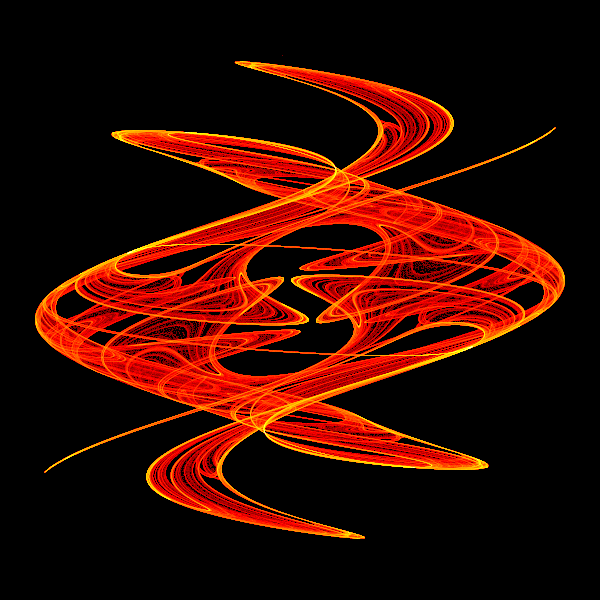# King's dream fractal in generativepy

Martin McBride
2021-12-15

The king's dream fractal works similarly to the Tinkerbell fractal. It is worth reading the tinkerbell fractal article, and the article on colorising tinkerbell before tackling the king's dream fractal in this article.

## Kings dream formula

The fractal equations for king's dream are:

xnext = math.sin(A*x)+B*math.sin(A*y)
ynext = math.sin(C*x)+D*math.sin(C*y)


Where:

A = 2.879879
B = -0.765145
C = -0.966918
D = 0.744728


Here is the image it creates:## The code

Here is the full code for the image above:

from generativepy.bitmap import Scaler
from generativepy.nparray import (make_nparray_data, save_nparray, load_nparray,
make_npcolormap, apply_npcolormap, save_nparray_image)
from generativepy.color import Color
from generativepy.utils import temp_file
import math
import numpy as np

MAX_COUNT = 10000000
A = 2.879879
B = -0.765145
C = -0.966918
D = 0.744728

def paint(image, pixel_width, pixel_height, frame_no, frame_count):
scaler = Scaler(pixel_width, pixel_height, width=4, startx=-2, starty=-2)

x = 2
y = 2
for i in range(MAX_COUNT):
x, y = math.sin(A*x)+B*math.sin(A*y), math.sin(C*x)+D*math.sin(C*y)
px, py = scaler.user_to_device(x, y)
image[py, px] += 1

def colorise(counts):
counts = np.reshape(counts, (counts.shape, counts.shape))
power_counts = np.power(counts, 0.25)
maxcount = np.max(power_counts)
normalised_counts = (power_counts * 1023 / max(maxcount, 1)).astype(np.uint32)

colormap = make_npcolormap(1024, [Color('black'), Color('red'), Color('orange'), Color('yellow'), Color('white')])

outarray = np.zeros((counts.shape, counts.shape, 3), dtype=np.uint8)
apply_npcolormap(outarray, normalised_counts, colormap)
return outarray

data = make_nparray_data(paint, 600, 600, channels=1)

filename = temp_file('kings-dream.dat')
save_nparray(filename, data)

frame = colorise(data)

save_nparray_image('kings-dream.png', frame)


This code is available on github in blog/fractals/kings_dream.py.

The code is very similar to the coloured Tinkerbell code, the main differences being:

• Different formulae in the calculation.
• Different parameters values A to D.
• Different Scaler arguments. The King's Dream fractal occupies a slight;y different space to the Tinkerbell.

## Variants

You can try different values of the constants. A and B need to be in the range -3 to +3, while C and D need to be in the range -1.5 to +1.5, otherwise, the values will fly off to infinity rather than creating a pattern.

Be aware that most numbers you choose will not create pleasing patterns. You will need to experiment to find something that looks nice and then do even more fine-tuning to get something really nice.

You can also try varying the function. You can replace sin with cos in some or all of the equations. This will give different but similar patterns.